# Chapter 3 Collisions in Plasmas

## 3.1  Binary collisions between charged particles

Reduced-mass for binary collisions:
Two particles interacting with each other have forces
F12 force on 1 from 2.
F21 force on 2 from 1.
By Newton's 3rd law, F12 = − F21.
Equations of motion:
 m1 ⋅⋅ r 1 = F12       ;       m2 ⋅⋅ r 2 = F21
(3.1)
Combine to get
 ⋅⋅ r 1 − ⋅⋅ r 2 = F12 ⎛⎝ 1 m1 + 1 m2 ⎞⎠
(3.2)
which may be written
 m1m2 m1 + m2 d2 dt2 (r1 − r2 ) = F12
(3.3)
If F12 depends only on the difference vector r1r2, then this equation is identical to the equation of a particle of "Reduced Mass" mr ≡ [(m1m2)/(m1 + m2 )] moving at position rr1r2 with respect to a fixed center of force:
 mr ⋅⋅ r = F12 (r)    .
(3.4)
This is the equation we analyse, but actually particle 2 does move. And we need to recognize that when interpreting mathematics.
If F21 and r1r2 are always parallel, then a general form of the trajectory can be written as an integral. To save time we specialize immediately to the Coulomb force
 F12 = q1 q2 4 πϵ0 r r3
(3.5)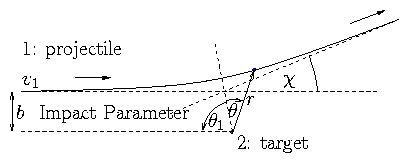Figure 3.1: Geometry of the collision orbit
Solution of this standard (Newton's) problem:
Angular momentum is conserved:
 mr r2 ⋅ θ =  const.   =  mr bv1       (θ clockwise  from  symmetry)
(3.6)
Substitute u ≡ 1/r  then  · θ = [(bv1)/(r2)] = u2  bv1
Also
 ⋅ r
 =
 d dt 1 u =  − 1 u2 du dθ ⋅ θ =  − bv1 du dθ
(3.7)
 ⋅⋅ r
 =
 − bv1 d2u dθ2 ⋅ θ =  − ( bv1)2  u2 d2 u dθ2
(3.8)
 ⋅⋅ r − r ⋅ θ 2 = − (bv1)2  u2 ⎛⎝ d2 u dθ2 + u ⎞⎠ = F12 mr
(3.9)
i.e.
 d2 u dθ2 + u = − q1 q2 4 πϵ0 1 mr ( bv1 )2
(3.10)
This orbit equation has the elementary solution
 u ≡ 1 r =  C cosθ − q1 q2 4 πϵ0 1 mr ( bv1 )2
(3.11)
The sinθ term is absent by symmetry. The other constant of integration, C, must be determined by initial condition. At initial (far distant) angle, θ1, u1 = [1/(∞)] = 0 . So
 0 = C cosθ1 − q1 q2 4 πϵ0 1 mr ( bv1 )2
(3.12)
There:
 ⋅ r 1 = − v1 = − bv1 du dθ |1 = + bv1 C sinθ1
(3.13)
Hence
tanθ1 = sinθ1

cosθ1
= −1 / Cb

 q1 q2 4 πϵ0 1 mr ( bv1 )2 / C
= − b

b90
(3.14)
where
 b90 ≡ q1 q2 4 πϵ0 1 mr v12 .
(3.15)
Notice that tanθ1 = −1 when b = b90. This is when θ1 = −45° and χ = 90°. So particle emerges at 90° to initial direction when
 b   =  b90              "90°  impact parameter"
(3.16)
Finally:
 C = − 1 b cosecθ1 = − 1 b ⎛⎝ 1 + b902 b2 ⎞⎠ 1/2
(3.17)

### 3.1.1  Frames of Reference

Key quantity we want is the scattering angle but we need to be careful about reference frames.
Most "natural" frame of ref is "Center-of-Mass" frame, in which C of M is stationary. C of M has position:
 R= m1 r1 + m2 r2 m1 + m2
(3.18)
and velocity (in lab frame)
 V = m1v1 + m2v2 m1 + m2
(3.19)
Now
 r1
 =
 R+ m2 m1 + m2 r
(3.20)
 r2
 =
 R− m1 m1 + m2 r
(3.21)
So motion of either particle in C of M frame is a factor times difference vector, r.
Velocity in lab frame is obtained by adding V to the C of M velocity, e.g. [(m2 · r)/(m1 + m2)] + V.
Angles of position vectors and velocity differences are same in all frames.
Angles (i.e. directions) of velocities are not same.

### 3.1.2  Scattering Angle

In C of M frame is just the final angle of r.
 − 2 θ1 + χ = π
(3.22)
1 is negative)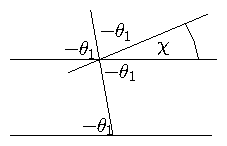Figure 3.2: Relation between θ1 and χ.

 χ = π+ 2 θ1    ;    θ1 = χ− π 2 .
(3.23)

 tanθ1 = tan ⎛⎝ χ 2 − π 2 ⎞⎠ = − cot χ 2
(3.24)
So
 cot χ 2
 =
 b b90
(3.25)
 tan χ 2
 =
 b90 b
(3.26)
But scattering angle (defined as exit velocity angle relative to initial velocity) in lab frame is different.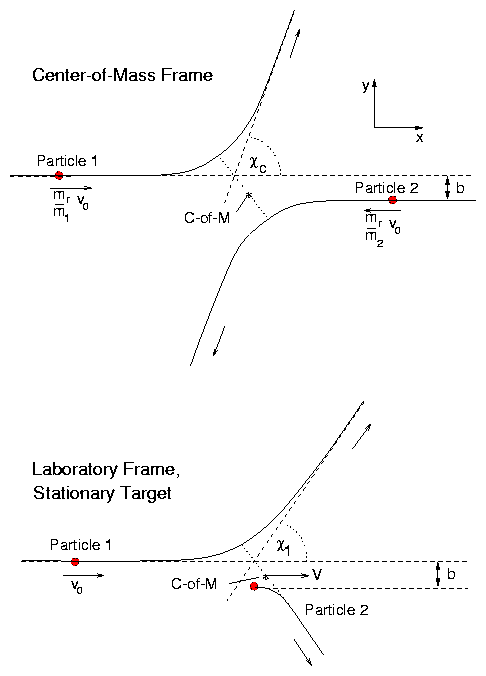Figure 3.3: Collisions viewed in Center of Mass and Laboratory frame.
Final velocity in CM frame
 v′CM
 =
 v1CM ( cosχc, sinχc ) = m2 m1 + m2 v1 ( cosχc, sinχc )
(3.27)
[ χc ≡ χ and v1 is initial relative velocity]. Final velocity in Lab frame
 v′L = v′CM + V= ⎛⎝ V + m2v1 m1 + m2 cosχc , m2v1 m1 + m2 sinχc ⎞⎠
(3.28)
So angle is given by
cotχL =
 V + m2 v1 m1 + m2 cosχc

 m2v1 m1 + m2 sinχc
=   V

v1
m1 + m2

m2
cosecχc + cotχc
(3.29)
For the specific case when m2 is initially a stationary target in lab frame, then
 V
 =
 m1v1 m1 + m2 and  hence
(3.30)
 cotχL
 =
 m1 m2 cosecχc + cotχc
(3.31)
This is exact.
Small angle approximation (cotχ→ [ 1/(χ)]) , cosecχ→ [ 1/(χ)] gives
 1 χL = m1 m2 1 χc + 1 χc ⇔  χL = m2 m1 + m2 χc
(3.32)
So small angles are proportional, with ratio set by the mass-ratio of particles.

## 3.2  Differential Cross-Section for Scattering by Angle

 Rutherford Cross-Section
By definition the cross-section, σ, for any specified collision process when a particle is passing through a density n2 of targets is such that the number of such collisions per unit path length is n2σ.
Sometimes a continuum of types of collision is considered, e.g. we consider collisions at different angles (χ) to be distinct. In that case we usually discuss differential cross-sections (e.g [(dσ)/(dχ)]) defined such that number of collisions in an (angle) element dχ per unit path length is n2 [(dσ)/(dχ)] dχ. [Note that [(dσ)/(dχ)] is just notation for a number. Some authors just write σ(χ), but I find that less clear.]
Normally, for scattering-angle discrimination we discuss the differential cross-section per unit solid angle:
 dσ dΩs .
(3.33)
This is related to scattering angle integrated over all azimuthal directions of scattering by: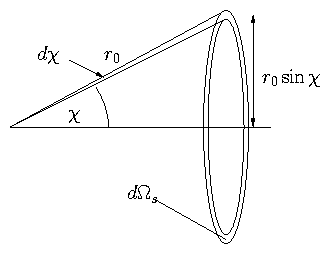Figure 3.4: Scattering angle and solid angle relationship.

 dΩs = 2 πsinχd χ
(3.34)
So that since
 dσ dΩs dΩs = dσ dχ dχ
(3.35)
we have
 dσ dΩs = 1 2 πsinχ dσ dχ
(3.36)
Now, since χ is a function (only) of the impact parameter, b, we just have to determine the number of collisions per unit length at impact parameter b.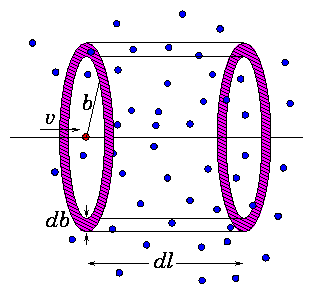Figure 3.5: Annular volume corresponding to db.
Think of the projectile as dragging along an annulus of radius b and thickness db for an elementary distance along its path, dl. It thereby drags through a volume:
 d l2 πb d b   .
(3.37)
Therefore in this distance it has encountered a total number of targets
 d l2 πb d b  .  n2
(3.38)
at impact parameter b(db). By definition this is equal to dl[(dσ)/db] db n2. Hence the differential cross-section for scattering (encounter) at impact parameter b is
 dσ db = 2 πb   .
(3.39)
Again by definition, since χ is a function of b
 dσ dχ dχ = dσ db db ⇒ dσ dχ = dσ db ⎢⎢ db dχ ⎢⎢ .
(3.40)
[db/dχ is negative but differential cross-sections are positive.]
Substitute and we get
 dσ dΩs = 1 2 πsinχ dσ db ⎢⎢ db dχ ⎢⎢ = b sinχ ⎢⎢ db dχ ⎢⎢ .
(3.41)
[This is a general result for classical collisions.]
For Coulomb collisions, in C of M frame,
 cot ⎛⎝ χ 2 ⎞⎠ = b b90
(3.42)

 ⇒ db dχ =  b90 d dχ cot χ 2 =  − b90 2 cosec2 χ 2 .
(3.43)
Hence
 d σ d Ωs
 =
 b90 cot χ 2

sinχ
b90

2
cosec2 χ

2
(3.44)
 =
b902

2

 cos χ 2 / sin χ 2

 2 sin χ 2 cos χ 2
1

 sin2 χ 2
(3.45)
 =
b902

 4 sin4 χ 2
(3.46)
This is the Rutherford Cross-Section.
d σ

d Ωs
= b902

 4 sin4 χ 2
(3.47)
for scattering by Coulomb forces through an angle χ measured in C of M frame.
Notice that [(dσ)/(dΩs)] → ∞ as χ→ 0.
This is because of the long-range nature of the Coulomb force. Distant collisions tend to dominate. (χ→ 0⇔b → ∞).

## 3.3  Relaxation Processes

There are 2 (main) different types of collisional relaxation process we need to discuss for a test particle moving through a background of scatterers:
1. Energy Loss (or equilibrium)
2. Momentum Loss (or angular scattering)
The distinction may be illustrated by a large angle (90°) scatter from a heavy (stationary) target.
If the target is fixed, no energy is transferred to it. So the energy loss is zero (or small if scatterer is just `heavy'). However, the momentum in the x direction is completely `lost' in this 90° scatter.
This shows that the timescales for Energy loss and momentum loss may be very different.

### 3.3.1  Energy Loss

For an initially stationary target, the final velocity in lab frame of the projectile is
 vL′ = ⎛⎝ m1v1 m1 + m2 + m2v1 m1 + m2 cosχc  , m2v1 m1 + m2 sinχc ⎞⎠
(3.48)
So the final kinetic energy is
 K′
 =
 1 2 m1vL′2 = 1 2 m1v12 ⎧⎨ ⎩ ⎛⎝ m1 m1 + m2 ⎞⎠ 2 + 2 m1m2 ( m1+m2 )2 cosχc
(3.49)
 + m22 ( m1 + m2 )2 ( cos2 χc + sin2 χc ) ⎫⎬ ⎭
(3.50)
 =
 1 2 m1v12 ⎧⎨ ⎩ 1 + 2m1m2 ( m1+m2 )2 ( cosχc − 1) ⎫⎬ ⎭
(3.51)
 =
 1 2 m1v12 ⎧⎨ ⎩ 1 − 2m1m2 ( m1+m2 )2 2 sin2 χc 2 ⎫⎬ ⎭
(3.52)
Hence the kinetic energy lost is ∆K = K − K′
 =
 1 2 m1v12 4 m1m2 ( m1+m2 )2 sin2 χc 2
(3.53)
 =
1

2
m1v12   4m1m2

( m1+m2 )2
1

 ⎛⎝ b b90 ⎞⎠ 2 + 1
[using  cot χc

2
= b

b90
]
(3.54)
(exact). For small angles χ << 1 i.e. b / b90 >> 1 this energy lost in a single collision is approximately
 ⎛⎝ 1 2 m1v12 ⎞⎠ 4 m1m2 ( m1+m2 )2 ⎛⎝ b90 b ⎞⎠ 2
(3.55)
If what we are asking is: how fast does the projectile lose energy? Then we need add up the effects of all collisions in an elemental length dl at all relevant impact parameters.
The contribution from impact parameter range db at b will equal the number of targets encountered times ∆K:

n2dl2 πb d b
encounters

 1 2 m1v12 4m1m2 ( m1+m2 )2 ⎛⎝ b90 b ⎞⎠ 2

Loss per encounter (∆K)

(3.56)
This must be integrated over all b to get total energy loss.
 dK = n2 dlK 4 m1m2 ( m1+m2 )2 ⌠⌡ ⎛⎝ b90 b ⎞⎠ 2 2 πb d b
(3.57)
so
 dK dl = K  n2 m1 m2 ( m1 + m2 )2 8 πb902  [ ln| b | ]maxmin
(3.58)
We see there is a problem both limits of the integral (b → 0, b → ∞) diverge logarithmically. That is because the formulas we are integrating are approximate.
1. We are using small-angle approx for ∆K.
2. We are assuming the Coulomb force applies but this is a plasma so there is screening.

### 3.3.2  Cut-offs Estimates

1. Small-angle approx breaks down around b = b90. Just truncate the integral there; ignore contributions from b < b90. Actually this apparently arbitrary approximation is rigorously justified by an integration of the exact (not small angle) loss expression.
 ⌠⌡ 2bdb (b/b90)2+1 = b902ln[(b/b90)2+1]     →b2→ 0     as b→0.
So if we had not made the small-angle approximation (which is here not mathematically essential) we would not have had a small-b divergence, we would have got zero from the lower limit.
2. Large-b cut-off arises because the Coulomb potential does not apply to arbitrarily large distances. Debye Shielding says really the potential varies as
ϕ ∝
 exp ⎛⎝ −r λD ⎞⎠

r

r
(3.59)
so approximate this by cutting off integral at b = λD equivalent to So the cut-offs can be taken as bmin   =  b90 and bmax = λD .
 dK dl
 =
 K n2 m1m2 (m1+m2 )2 8 πb902  ln| Λ |
(3.60)
 Λ
 =
 λD b90 = ⎛⎝ ϵ0 Te n e2 ⎞⎠ 1/2 / ⎛⎝ q1q2 4 πϵ0 mrv12 ⎞⎠
(3.61)
So Coulomb Logarithm is `lnΛ'
 Λ   = λD b90 = ⎛⎝ ϵ0 Te n e2 ⎞⎠ 1/2 / ⎛⎝ q1q2 4 πϵ0 mrv12 ⎞⎠
(3.62)
Because these cut-offs are in ln term result is not sensitive to their exact values.
One commonly uses Collision Frequency. Energy Loss Collision Frequency is
 νK ≡ v1 1 K dK dl = n2 v1 m1m2 ( m1 + m2 )2 8 πb902  ln| Λ |
(3.63)
Substitute for b90 and mr (in b90)
 νK
 =
n2 v1   m1m2

( m1+m2 )2
8 π

q1q2

 4 πϵ0 m1m2 m1+m2 v12

2

lnΛ
(3.64)
 =
 n2 q12q22 ( 4 πϵ0 )2 8 π m1m2v13 lnΛ
(3.65)
Collision time    τK ≡ 1/νK
Effective (Energy Loss) Cross-section      [ 1/K [dK/(d l)] = σKn2 ]
 σK = νK / n2v1 = q12q22 ( 4 πϵ0 )2 8 π m1m2v14 lnΛ.
(3.66)

### 3.3.3  Momentum Loss

Loss of x-momentum in 1 collision is
 ∆px
 =
 m1 (v1 − vLx′)
(3.67)
 =
 m1v1 ⎧⎨ ⎩ 1 − ⎛⎝ m1 m1+m2 + m2 m1+m2 cosχc ⎞⎠ ⎫⎬ ⎭
(3.68)
 =
 px m2 m1+m2 ( 1 − cosχc )
(3.69)
 ≅
 px m2 m1+m2 χc2 2 = px m2 m1+m2 2 b902 b2
(3.70)
(small angle approx). Hence rate of momentum loss can be obtained using an integral identical to the energy loss but with the above parameters:
 dp dl
 =
 n2p ⌠⌡ bmax bmin m2 m1 + m2 2 b902 b2 2 πb d b
(3.71)
 =
 n2 p m2 m1 + m2 4π b902  lnΛ
(3.72)
Note for future reference:
 dp dt = v1 dp dl = n2v12 m1m2 m1 + m2 4 πb902  lnΛ .
(3.73)
Therefore Momentum Loss.
Collision Frequency
 νp
 =
 v1 1 p dp dl = n2v1 m2 m1+m2 4 πb902 lnΛ
(3.74)
 =
n2v1   m2

m1+m2
4 π

q1q2

 4 πϵ0 m1m2 m1+m2 v12

2

lnΛ
(3.75)
 =
 n2 q12q22 ( 4 πϵ0 )2 4 π(m1+m2) m2m12v13 lnΛ
(3.76)
Collision Time      τp = 1/νp
Cross-Section (effective)      σ = νp/n2v1
Notice ratio
 Energy Loss νK Momentum loss νp = 2 m1m2 / m1+m2 m2m12 = 2 m1 m1 + m2
(3.77)
This is
 ≅
 2     if     m1 >> m2
(3.78)
 =
 1    if     m1 = m2
(3.79)
 <<
 1     if     m1 << m2.
(3.80)
Third case, e.g. electrons → ions shows that mostly the angle of velocity scatters. Therefore Momentum `Scattering' time is often called `90° scattering' time to `diffuse' through 90° in angle.

### 3.3.4  `Random Walk' in angle

When m1 << m2, energy loss << momentum loss. Hence |vL′| ≅ v1. All that matters is the scattering angle: χL ≅ χc ≅ 2 b90/b.
Mean angle of total deviation ∆α in length L is zero because all directions are equally likely.
But:
Mean square angle is
 ∆α2
 =
 n2 L ⌠⌡ bmax bmin χ2  2 πb d b
(3.81)
 =
 L n2  8 π b902  lnΛ
(3.82)
Spread is `all round' when ∆α2 ≅ 1. This is roughly when a particle has scattered 90° on average. It requires
 L n2  8 πb902  lnΛ = 1   .
(3.83)
So can think of a kind of `cross-section' `σ90' for 90° scattering as such that
 n2 L `σ90′
 =
 1  when  L n2  8 π b902  lnΛ = 1
(3.84)
 i.e.  `σ90′
 =
 πb902 8 lnΛ ( = 2 σp)
(3.85)
This is 8 lnΛ larger than cross-section for 90° scattering in single collision.
Be Careful!90' is not a usual type of cross-section because the whole process is really diffusive in angle.
Actually all collision processes due to coulomb force are best treated (in a Mathematical way) as a diffusion in velocity space
→ Fokker-Planck equation.

### 3.3.5  Summary of different types of collision

The Energy Loss collision frequency is to do with slowing down to rest and exchanging energy. It is required for calculating
Equilibration Times (of Temperatures)
Energy Transfer between species.
The Momentum Loss frequency is to do with loss of directed velocity. It is required for calculating
Mobility: Conductivity/Resistivity
Viscosity
Particle Diffusion
Energy (Thermal) Diffusion
Usually we distinguish between electrons and ions because of their very different mass:
Energy Loss      [Stationary Targets]       Momentum Loss

 Kνee
 =
 ne e4 ( 4 πϵ0 )2 8 π me2 ve3 lnΛ         pνee =  Kνee × ⎡⎣ me+me 2me = 1 ⎤⎦
 Kνei
 =
 ni Z2e4 ( 4 πϵ0 )2 8 π memive3 lnΛ      pνei =  Kνei × ⎡⎣ me+mi 2me ≅ mi 2me ⎤⎦
 Kνii
 =
 ni Z2e4 ( 4 πeo )2 8 π mi2vi3 lnΛ         pνii =  Kνii × ⎡⎣ mi+mi 2mi = 1 ⎤⎦
(3.86)
 Kνie
 =
 ne Ze2e4 ( 4 πϵ0 )2 8 π mimevi3 lnΛ      pνie =  Kνie × ⎡⎣ me+mi 2mi ≅ 1 2 ⎤⎦
Sometimes one distinguishes between `transverse diffusion' of velocity and `momentum loss'. The ratio of these two is
 ∆p⊥2

p2l
/
∆p||

p ∆l

 =
 d χL2 d l / ⎢⎢ 1 p dp dl ⎢⎢
(3.87)
 =
 ⎛⎝ m2 m1 + m2 χc ⎞⎠ 2

 m2 m1+m2 χc2 2
= 2m2

m1+m2
.
(3.88)
So
 `σ90′ `σp′ = 2 m2 m1+m2
 =
 1        like particles
(3.89)
 ≅
 2         m1 << m2
(3.90)
 ≅
 2m2 m1 m2 << m1 .
(3.91)
Hence

 ⊥νee
 =
 pνee =  Kνee ( = `νee′!!)
(3.92)
 ⊥νei
 =
 2 pνei =  Kνee ni ne Z2 ( = Zνee ) ( = `νei′)
(3.93)
 ⊥νii
 =
 pνii =  Kνii ( = νii!! )  (Like Ions)
(3.94)
 ⊥νie
 =
 2me mi pνie = me mi Kνie =  Kνii = νii
(3.95)
[But note: ions are slowed down by electrons long before being angle scattered.]

## 3.4  Thermal Distribution Collisions

So far we have calculated collision frequencies with stationary targets and single-velocity projectiles but generally we shall care about thermal (Maxwellian) distributions (or nearly thermal) of both species. This is harder to calculate and we shall resort to some heuristic calculations.

### 3.4.1  e → i

Very rare for thermal ion velocity to be  ∼  electron. So ignore ion motion.
Average over electron distribution.
Momentum loss to ions from (assumed) drifting Maxwellian electron distribution:
 fe (v) = ne ⎛⎝ me 2 πTe ⎞⎠ 3/2 exp ⎡⎣ − m( v− vd )2 2T ⎤⎦
(3.96)
Each electron in this distribution is losing momentum to the ions at a rate given by the collision frequency
 νp = ni qe2qi2 ( 4 πϵ0 )2 4 π( me+mi ) mime2v3 lnΛ
(3.97)
so total rate of loss of momentum is given by (per unit volume)
 − dp dt = ⌠⌡ fe(v)  νp(v)  mev d3v
(3.98)
To evaluate this integral approximately we adopt the following simplifications.
1. Ignore variations of lnΛ with v and just replace a typical thermal value in Λ = λD/b90(v1).
2. Suppose that drift velocity vd is small relative to the typical thermal velocity, written ve ≡ √{Te/me} and express fe in terms of u ≡ [(v)/(ve)] to first order in ud ≡ [(vd)/(ve)]:
 fe
 =
 ne 1 ( 2 π)3/2 ve3 exp ⎡⎣ −1 2 ( u − ud )2 ⎤⎦
(3.99)
 ≅
 ne ( 2π)3/2 ve3 ( 1 + u . ud )  exp ⎡⎣ −u2 2 ⎤⎦ = ( 1 + ux ud ) fo
(3.100)
taking x-axis along ud and denoting by fo the unshifted Maxwellian.
Then momentum loss rate per unit volume
 − dpx dt
 =
 ⌠⌡ fe νp mevx d3 v
 =
 νp (ve) me ⌠⌡ (1 + ux ud) fo ve3 v3 vx  d3  v
(3.101)
 =
 νp (ve) mevd ⌠⌡ ux2 u3 fo  d3 v
To evaluate this integral, use the spherical symmetry of fo to see that:
 ⌠⌡ ux2 u3 fo d3 v
 =
 1 3 ⌠⌡ ux2 + uy2 + uz2 u3 fo d3 v= 1 3 ⌠⌡ u2 u3 fo d3 v
 =
 1 3 ⌠⌡ α 0 ve v fo 4 πv2 d v
 =
 2 π 3 ve ⌠⌡ α 0 fo 2 v d v
 =
 2 π 3 ve ne ( 2 π)3/2 ve3 ⌠⌡ α 0 exp ⎛⎝ −v2 2ve2 ⎞⎠ dv2
 =
 2 π 3 ne ( 2 π)3/2 2 = 2 3 ( 2 π)1/2 ne  .
(3.102)
Thus the Maxwell-averaged momentum-loss frequency is
 − 1 p dp dt ≡ ν ei = 2 3 ( 2 π)1/2 νp (ve)
(3.103)
(where p = mevdne is the momentum per unit volume attributable to drift).
 ν ei
 =
 2 3 ( 2 π)1/2 ni qe2qi2 ( 4 πϵ0 )2 4 π( me+mi ) mi me2 ve3 lnΛe
(3.104)
 =
 2 3 ( 2 π)1/2 ni ⎛⎝ Ze2 4 πϵ0 ⎞⎠ 2 4 π me1/2 Te3/2 lnΛe
(3.105)
(substituting for thermal electron velocity, ve, and dropping [(me)/(mi)] order term), where Ze = qi.
This is the standard form of electron collision frequency.

### 3.4.2  i → e

Ion momentum loss to electrons can be treated by a simple Galilean transformation of the e → i case because it is still the electron thermal motions that matter.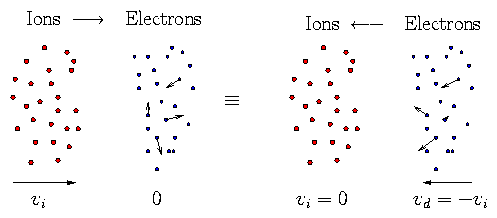Figure 3.6: Ion-electron collisions are equivalent to electron-ion collisions in a moving reference frame.
Rate of momentum transfer, [dp/dt], is same in both cases:
 dp dt = − p ν
(3.106)
Hence |peei = |pi| νie or
 ν ie = |pe| |pi| ν ei = neme nimi ν ei
(3.107)
(since drift velocities are the same).
Ion momentum loss to electrons has much lower collision frequency than e → i because ions possess so much more momentum for the same velocity.

### 3.4.3  i → i

Ion-ion collisions can be treated somewhat like e → i collisions except that we have to account for moving targets i.e. their thermal motion.
Consider two different ion species moving relative to each other with drift velocity vd; the targets' thermal motion affects the momentum transfer cross-section.
Using our previous expression for momentum transfer, we can write the average rate of transfer per unit volume as: [see 3.73 "note for future reference"]
 − dp dt = ⌠⌡ ⌠⌡ vr m1m2 m1+m2 vr 4 π b902  lnΛ  f1f2 d3 v1 d3 v2
(3.108)
where vr is the relative velocity (v1v2) and b90 is expressed
 b90 = q1q2 4 πϵ0 1 mrvr2
(3.109)
and mr is the reduced mass [(m1m2)/(m1+m2)].
Since everything in the integral apart from f1f2 depends only on the relative velocity, we proceed by transforming the velocity coordinates from v1,v2 to being expressed in terms of relative (vr) and average (i.e. individual center of mass velocity, V)
 vr ≡ v1 − v2      ;      V ≡ m1v1 + m2v2 m1+m2 .
(3.110)
Take f1 and f2 to be shifted Maxwellians in the overall C of M frame:
 fj = nj ⎛⎝ mj 2 πT ⎞⎠ 3/2 exp ⎡⎣ − mj ( vj − vdj )2 2 T ⎤⎦ (j = 1, 2)
(3.111)
where m1vd1 + m2vd2 = 0. Then
 f1f2
 =
 n1n2 ⎛⎝ m1 2πT ⎞⎠ 3/2 ⎛⎝ m2 2 πT ⎞⎠ 3/2 exp ⎡⎣ − m1v12 2 T − m2v22 2T ⎤⎦
 × ⎧⎨ ⎩ 1 + v1 . m1vd1 T + v2 . m2vd2 T ⎫⎬ ⎭
(3.112)
to first order in vd. Convert to local CM v-coordinates and find (after algebra)
 f1f2
 =
 n1n2 ⎛⎝ M 2πT ⎞⎠ 3/2 ⎛⎝ mr 2πT ⎞⎠ 3/2 exp ⎡⎣ − MV2 2T − mrvr2 2T ⎤⎦
 × ⎧⎨ ⎩ 1 + mr T vd . vr ⎫⎬ ⎭
(3.113)
where M = m1+m2. Note also that (it can be shown) that the Jacobian of the transformation is unity d3v1 d3v2 = d3vrd3V. Hence
 − dp dt
 =
 ⌠⌡ ⌠⌡ vr mr vr 4 π b902lnΛ n1n2 ⎛⎝ M 2πT ⎞⎠ 3/2 ⎛⎝ mr 2πT ⎞⎠ 3/2
 exp ⎛⎝ − MV2 2T ⎞⎠ exp ⎛⎝ − mrvr2 2T ⎞⎠ ⎧⎨ ⎩ 1 + mr T vd . vr ⎫⎬ ⎭ d3 vr d3 V
(3.114)
and since nothing except the exponential depends on V, that integral can be done:
 − dp dt = ⌠⌡ vr mrvr4πb902 lnΛ n1n2 ⎛⎝ mr 2πT ⎞⎠ 3/2 exp ⎛⎝ −mrvr2 2π ⎞⎠ ⎧⎨ ⎩ 1 + mr T vd . vr ⎫⎬ ⎭ d3 vr
(3.115)
This integral is of just the same type as for e−i collisions, i.e.
 − dp dt
 =
 vd vri mr  4 π b902 (vri) lnΛt  n1n2 ⌠⌡ ux2 u3 ^ f o (vr) d3 vr
 =
 vdvri mr 4  π b902 (vri)  ln Λt n1n2 2 3 ( 2π)1/2
(3.116)
where vri ≡ √{[T/(mr)]}, b902 (vri) is the ninety degree impact parameter evaluated at velocity vtr, and fo is the normalized Maxwellian.
 − dp dt = 2 3 ( 2π)1/2 ⎛⎝ q1q2 4 πϵ0 ⎞⎠ 2 4 π mr2vri3 lnΛt  n1n2 mr vd
(3.117)
This is the general result for momentum exchange rate between two Maxwellians drifting at small relative velocity vd.
To get a collision frequency is a matter of deciding which species is stationary and so what the momentum density of the moving species is. Suppose we regard 2 as targets then momentum density is n1m1vd so
 ν 12 = 1 n1m1vd dp dt = 2 3 ( 2 π)1/2 n2 ⎛⎝ q1q2 4 πϵ0 ⎞⎠ 2 4 π mr vri3 lnΛt m1 .
(3.118)
This expression works immediately for electron-ion collisions substituting mr ≅ me, recovering previous.
For equal-mass ions mr = [(mi2)/(mi+mi)] = 1/2 mi and vri = √{[T/(mr)]} = √{[2T/(mi)]}.
Substituting, we get
 ν ii = 1 3 π1/2 ni ⎛⎝ q1q2 4 πϵ0 ⎞⎠ 2 4 π mi1/2 Ti3/2 lnΛ
(3.119)
that is, [ 1/(√2 )] times the e−i expression but with ion parameters substituted. [Note, however, that we have considered the ion species to be different.]

### 3.4.4  e → e

Electron-electron collisions are covered by the same formalism, so
 ν ee = 1 3 π1/2 ne ⎛⎝ e2 4 πϵ0 ⎞⎠ 2 4 π me1/2 Te3/2 lnΛ   .
(3.120)
However, the physical case under discussion is not so obvious; since electrons are indistiguishable how do we define two different "drifting maxwellian" electron populations? A more specific discussion would be needed to make this rigorous.
Generally νee  ∼ νei/ √2 : electron-electron collision frequency  ∼  electron-ion (for momentum loss).

### 3.4.5  Summary of Thermal Collision Frequencies

For momentum loss:
 ν ei
 =
√2

 3 √ π
ni
Ze2

4 πϵ0

2

4 π

me1/2 Te3/2
lnΛe   .
(3.121)
 ν ee
 ≅
 1 √2 ν ei .     (electron parameters)
(3.122)
 ν ie
 =
 neme nimi ν ei .
(3.123)
 ν ii′
 =
√2

 3 √ π
ni′
qiqi′

4 πϵ0

2

4 π

mi1/2 Ti3/2

mi′

mi+mi′

1/2

lnΛi
(3.124)
Energy loss Kν related to the above (pν) by
 Kν = 2m1 m1+m2 pν  .
(3.125)
Transverse `diffusion' of momentum ν, related to the above by:
 ⊥ ν = 2m2 m1+m2 pν  .
(3.126)

## 3.5  Applications of Collision Analysis

### 3.5.1  Energetic (`Runaway') Electrons

Consider an energetic (1/2 me v12 >> T) electron travelling through a plasma. It is slowed down (loses momentum) by collisions with electrons and ions (Z), with collision frequency:
 pνee
 =
 νee = ne e4 ( 4 πϵ0)2 8 π me2 v13 lnΛ
(3.127)
 pνei
 =
 ni 2ne Z2  νee = Z 2 νee
(3.128)
Hence (in the absence of other forces)
 d dt (mev)
 =
 − ( pνee +  pνei ) mp v
(3.129)
 =
 − ⎛⎝ 1 + Z 2 ⎞⎠ νee  mev
(3.130)
This is equivalent to saying that the electron experiences an effective `Frictional' force
 Ff
 =
 d dt (mev) = − ⎛⎝ 1 + Z 2 ⎞⎠ νee  me v
(3.131)
 Ff
 =
 − ⎛⎝ 1 + Z 2 ⎞⎠ ne e4 ( 4 πϵ0 )2 8 π lnΛ mev2
(3.132)
Notice
1. for Z=1 slowing down is 2/3 on electrons 1/3 ions
2. Ff decreases with v increasing.
Suppose now there is an electric field, E. The electron experiences an accelerating Force.
Total force
 F = d dt (mv) = −eE + Ff = −eE − ⎛⎝ 1 + Z 2 ⎞⎠ ne e4 ( 4 πϵ0 )2 8 πlnΛ mev2
(3.133)
Two Cases   (When E is accelerating)
1. | eE | < | Ff |: Electron Slows Down
2. | eE | > | Ff |: Electron Speeds Up!
Once the electron energy exceeds a certain value its velocity increases continuously and the friction force becomes less and less effective. The electron is then said to have become a `runaway'.
Condition:
 1 2 mev2 > ⎛⎝ 1 + Z 2 ⎞⎠ ne e4 ( 4 πϵ0 )2 8 πlnΛ 2 e E
(3.134)

### 3.5.2  Plasma Resistivity (DC)

Consider a bulk distribution of electrons in an electric field. They tend to be accelerated by E and decelerated by collisions.
In this case, considering the electrons as a whole, no loss of total electron momentum by e−e collisions. Hence the friction force we need is just that due to νei.
If the electrons have a mean drift velocity vd ( << vthe) then
 d dt (mevd) = − e E − ν ei me vd
(3.135)
vd = − e E

 me ν ei
.
(3.136)
The current density is then
j = − ne e vd = ne e2 E

 me ν ei
(3.137)
Now generally, for a conducting medium we define the conductivity, σ, or resistivity, η, by
 j = σE    ;    ηj = E ⎛⎝ σ = 1 η ⎞⎠
(3.138)
Therefore, for a plasma,
σ = 1

η
= ne e2

 me ν ei
(3.139)
Substitute the value of νei and we get
 η
 ≅
ni Z2

ne
· e2 me1/2  8 π lnΛ

 ( 4 πϵ0 )2  3 √ 2 π Te3/2
(3.140)
 =
Z e2 me1/2  8 π lnΛ

 ( 4 πϵ0 )2  3 √ 2 π Te3/2
(for a single ion species).
(3.141)
Notice
1. Density cancels out because more electrons means (a) more carriers but (b) more collisions.
2. Main dependence is η ∝ Te−3/2. High electron temperature implies low resistivity (high conductivity).
3. This expression is only approximate because the current tends to be carried by the more energetic electrons, which have smaller νei; thus if we had done a proper average over f(ve) we expect a lower numerical value. Detailed calculations give
 η = 5.2 ×10−5 lnΛ ( Te/eV )3/2 Ωm
(3.142)
for Z = 1 (vs. our expression ≅ 10−4). This is `Spitzer' resistivity. The detailed calculation value is roughly a factor of two smaller than our calculation, which is not a negligible correction!

### 3.5.3  Diffusion

For motion parallel to a magnetic field if we take a typical electron, with velocity v|| ≅ vte it will travel a distance approximately
 le = vte / ν ei
(3.143)
before being pitch-angle scattered enough to have its velocity randomised. [This is an order-of-magnitude calculation so we ignore νee.] l is the mean free path.
Roughly speaking, any electron does a random walk along the field with step size l and step frequency νei. Thus the diffusion coefficient of this process is
De||le2

ν

ei
vte2

 ν ei
∼  T5/2

n
.
(3.144)
Similarly for ions
Di||li2

ν

ii
vti2

 ν ii
∼  T5/2

n
(3.145)
Notice
 ν ii / ν ei ≅ ⎛⎝ me mi ⎞⎠ 1/2 ≅ vti vte (if  Te ≅ Ti)
(3.146)
Hence         leli
Mean free paths for electrons and ions are  ∼  same.
The diffusion coefficients are in the ratio
 Di De ≅ ⎛⎝ me mi ⎞⎠ 1/2 :   Ions diffuse slower in parallel direction.
(3.147)
Diffusion Perpendicular   to Mag. Field is different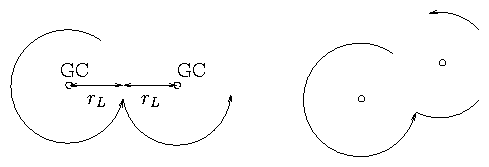Figure 3.7: Cross-field diffusion by collisions causing a jump in the gyrocenter (GC) position.
Roughly speaking, if electron direction is changed by  ∼ 90° the Guiding Centre moves by a distance  ∼ rL. We may think of this as a random walk with step size  ∼ rL and frequency νei. Hence
 De⊥ ≅ rLe2 ν ei ≅ vte2 Ωe2 ν ei
(3.148)
Ion transport is similar but both require a discussion of the effects of like and unlike collisions.
Particle transport occurs only via unlike collisions. To show this we consider in more detail the change in guiding center position at a collision. Recall m· v = q vB which leads to
 v⊥ = q m rL ∧B        (perp. velocity only).
(3.149)
This gives
 rL = B∧mv⊥ q B2
(3.150)
At a collision the particle position does not change (instantaneously) but the guiding center position (r0) does.
 r0′+ rL′ = r0 + rL ⇒ ∆r0 ≡ r0′− r0 = − (rL′− rL)
(3.151)
Change in rL is due to the momentum change caused by the collision:
 rL′− rL = B q B2 ∧m(v′⊥ − v⊥) ≡ B q B2 ∧∆(m v⊥)
(3.152)
So
 ∆ r0 = − B q B2 ∧∆(m v⊥).
(3.153)
The total momentum conservation means that ∆(mv) for the two particles colliding is equal and opposite. Hence, from our equation, for like particles, ∆r0 is equal and opposite. The mean position of guiding centers of two colliding like particles (r01 + r02)/2 does not change.
No net cross field particle (guiding center) shift.
Unlike collisions (between particles of different charge q) do produce net transport of particles of either type. And indeed may move r01 and r02 in same direction if they have opposite charge.
 Di⊥ ≅ rLi2 pν ie ≅ vti2 Ωi2 pν ie
(3.154)
Notice that rLi2 / rLe2 ≅ mi/me    ;    pνie /νei ≅ [(me)/(mi)]
So Di⊥/De⊥ ≅ 1 (for equal temperatures). Collisional diffusion rates of particles are same for ions and electrons.
However energy transport is different because it can occur by like-like collisions.
Thermal Diffusivity:
 χe
 ∼
 rLe2 ⎛⎝ ν ei + ν ee ⎞⎠ ∼ rLe2 ν ei ⎛⎝ ν ei ∼ ν ee ⎞⎠
(3.155)
 χi
 ∼
 rLi2 ⎛⎝ pν ie + ν ii ⎞⎠ ≅ rLi2 ν ii ⎛⎝ ν ii >> ν ie ⎞⎠
(3.156)
 χi/χe
 ∼
rLi2

rLe2

 ν ii

 ν ei
mi

me
me1/2

mi1/2
=
mi

me

1/2

(equal T)
(3.157)
Collisional Thermal transport by Ions is greater than by electrons [factor  ∼ (mi/me)1/2 ].

### 3.5.4  Energy Equilibration

If Te ≠ Ti then there is an exchange of energy between electrons and ions tending to make Te = Ti. As we saw earlier
 Kνei = 2me mi pνei = me mi ⊥ νei
(3.158)
So applying this to averages.
 Kν ei ≅ 2me mi ν ei ( ≅ ν ie )
(3.159)
Thermal energy exchange occurs  ∼ me/mi slower than momentum exchange. (Allows Te ≠ Ti). So
 dTe dt = − dTi dt = − Kν ei ( Te − Ti )
(3.160)
From this one can obtain the heat exchange rate (per unit volume), Hei, say:
 Hei
 =
 − d dt ⎛⎝ 3 2 neTe ⎞⎠ = d dt ⎛⎝ 3 2 niTi ⎞⎠
(3.161)
 =
 − 3 4 n d dt ( Te − Ti ) = 3 4 n Kν ei ( Te − Ti )
(3.162)
Important point:
 Kν ei = 2me mi pνei = 2me mi √2Z Kνee ∼ 1 Z2 ⎛⎝ me mi ⎞⎠ 1/2 Kν ii .
(3.163)
`Electrons and Ions equilibrate among themselves much faster than with each other'.

## 3.6  Some Orders of Magnitude

1. lnΛ is very slowly varying. Typically has value  ∼  12 to 16 for laboratory plasmas.
2. νei ≈ 6 ×10−11 ( ni/m3 )/ ( Te/eV )3/2       (lnΛ = 15, Z = 1).
e.g. = 2 ×105 s−1 (when n = 1020m−3 and Te = 1 keV.) For phenomena which happen much faster than this, i.e. τ << 1 / νei  ∼ 5 μs, collisions can be ignored.
Examples: Electromagnetic Waves with high frequency.
3. Resistivity. Because most of the energy of a current carrying plasma is in the B field not the K.E. of electrons, resistive decay of current can be much slower than νei. E.g. Coaxial Plasma: (Unit length)
Inductance    L = μo  lnb/a
Resistance    R = η 1 / πa2
L/R decay time
 τR
 ∼
μo πa2

η
ln b

a
nee2

 me ν ei
μo  πa2 ln b

a
 ∼
nee2

meϵ0
a2

c2
1

 ν ei
= ωp2 a2

c2
· 1

 ν ei
>> 1

 ν ei
.
(3.164)
Comparison   1 keV temperature plasma has  ∼  same (conductivity/) resistivity as a slab of copper ( ∼ 2 ×10−8 Ωm).
Ohmic Heating   Because η ∝ Te−3 / 2, if we try to heat a plasma Ohmically, i.e. by simply passing a current through it, this works well at low temperatures but its effectiveness falls off rapidly at high temperature.
Result for most Fusion schemes it looks as if Ohmic heating does not quite yet get us to the required ignition temperature. We need auxilliary heating, e.g. Neutral Beams. (These slow down by collisions.)